Research papers and code for "Eugene Santos Jr":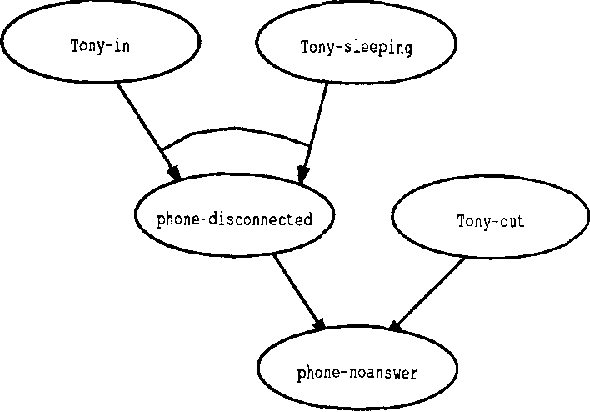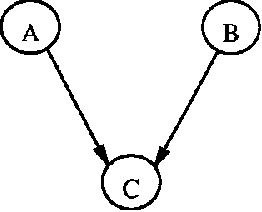In general, the best explanation for a given observation makes no promises on how good it is with respect to other alternative explanations. A major deficiency of message-passing schemes for belief revision in Bayesian networks is their inability to generate alternatives beyond the second best. In this paper, we present a general approach based on linear constraint systems that naturally generates alternative explanations in an orderly and highly efficient manner. This approach is then applied to cost-based abduction problems as well as belief revision in Bayesian net works.

* Appears in Proceedings of the Seventh Conference on Uncertainty in Artificial Intelligence (UAI1991)
Click to Read Paper and Get Code
Independence-based (IB) assignments to Bayesian belief networks were originally proposed as abductive explanations. IB assignments assign fewer variables in abductive explanations than do schemes assigning values to all evidentially supported variables. We use IB assignments to approximate marginal probabilities in Bayesian belief networks. Recent work in belief updating for Bayes networks attempts to approximate posterior probabilities by finding a small number of the highest probability complete (or perhaps evidentially supported) assignments. Under certain assumptions, the probability mass in the union of these assignments is sufficient to obtain a good approximation. Such methods are especially useful for highly-connected networks, where the maximum clique size or the cutset size make the standard algorithms intractable. Since IB assignments contain fewer assigned variables, the probability mass in each assignment is greater than in the respective complete assignment. Thus, fewer IB assignments are sufficient, and a good approximation can be obtained more efficiently. IB assignments can be used for efficiently approximating posterior node probabilities even in cases which do not obey the rather strict skewness assumptions used in previous research. Two algorithms for finding the high probability IB assignments are suggested: one by doing a best-first heuristic search, and another by special-purpose integer linear programming. Experimental results show that this approach is feasible for highly connected belief networks.

* Appears in Proceedings of the Tenth Conference on Uncertainty in Artificial Intelligence (UAI1994)
Click to Read Paper and Get Code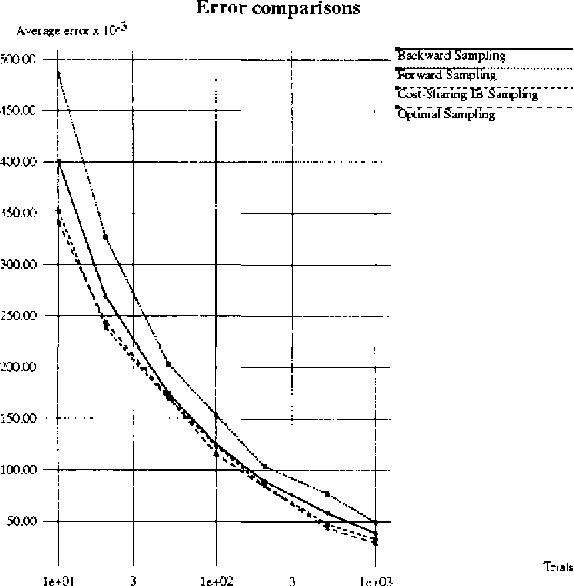Belief updating in Bayes nets, a well known computationally hard problem, has recently been approximated by several deterministic algorithms, and by various randomized approximation algorithms. Deterministic algorithms usually provide probability bounds, but have an exponential runtime. Some randomized schemes have a polynomial runtime, but provide only probability estimates. We present randomized algorithms that enumerate high-probability partial instantiations, resulting in probability bounds. Some of these algorithms are also sampling algorithms. Specifically, we introduce and evaluate a variant of backward sampling, both as a sampling algorithm and as a randomized enumeration algorithm. We also relax the implicit assumption used by both sampling and accumulation algorithms, that query nodes must be instantiated in all the samples.

* Appears in Proceedings of the Twelfth Conference on Uncertainty in Artificial Intelligence (UAI1996)
Click to Read Paper and Get Code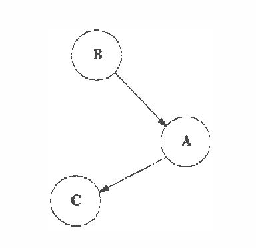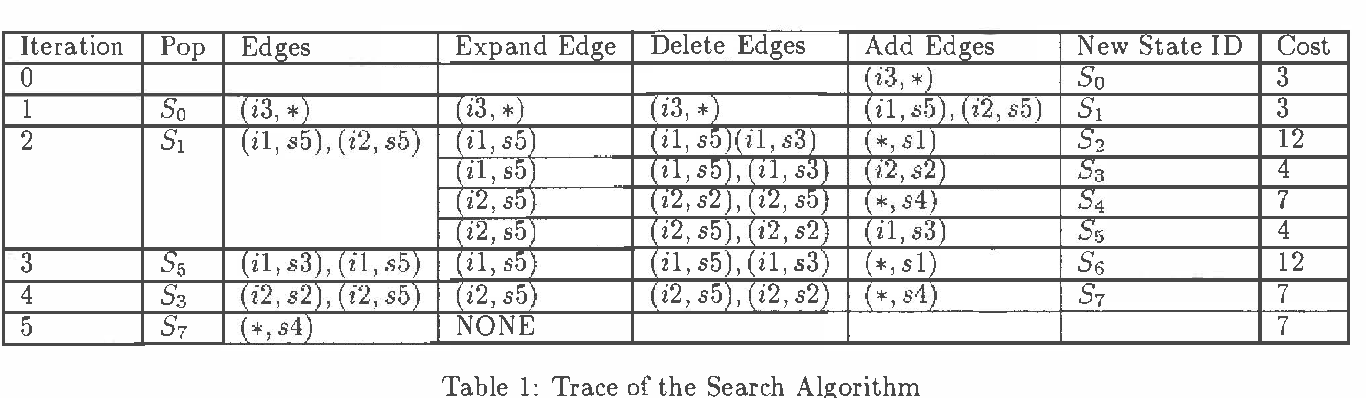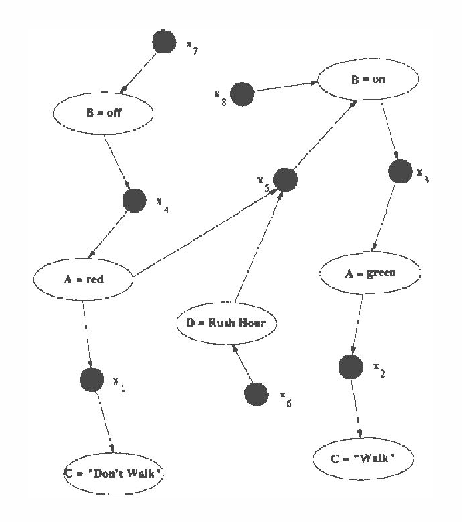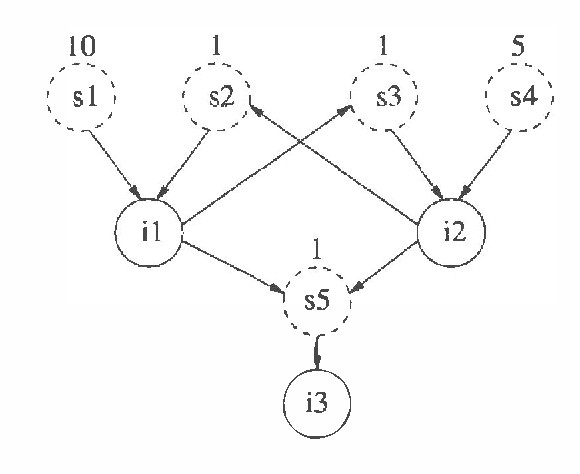Bayesian knowledge bases (BKBs) are a generalization of Bayes networks and weighted proof graphs (WAODAGs), that allow cycles in the causal graph. Reasoning in BKBs requires finding the most probable inferences consistent with the evidence. The cost-sharing heuristic for finding least-cost explanations in WAODAGs was presented and shown to be effective by Charniak and Husain. However, the cycles in BKBs would make the definition of cost-sharing cyclic as well, if applied directly to BKBs. By treating the defining equations of cost-sharing as a system of equations, one can properly define an admissible cost-sharing heuristic for BKBs. Empirical evaluation shows that cost-sharing improves performance significantly when applied to BKBs.

* Appears in Proceedings of the Thirteenth Conference on Uncertainty in Artificial Intelligence (UAI1997)
Click to Read Paper and Get Code